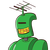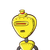# Sum of the areas of two squares is 468 m square if the difference of their perimeter is 24 m find the sides of the two square .​

Sum of the areas of two squares is 468 m square if the difference of their perimeter is 24 m find the sides of the two square .​

### 2 thoughts on “Sum of the areas of two squares is 468 m square if the difference of their perimeter is 24 m find the sides of the two square .​”

1.## ❥คɴᎦᴡєя

Let the sides of the first and second square be X and Y .

Area of the first square = (X)²

Area of the second square = (Y)²

According to question,

(X)² + (Y)² = 468 m² ——(1).

Perimeter of first square = 4 × X and Perimeter of second square = 4 × Y

According to question,

4X – 4Y = 24 ——–(2)

From equation (2) we get,

4X – 4Y = 244X–4Y=24

4(X-Y) = 244(X−Y)=24

X – Y = 24/4X–Y=24/4

X – Y = 6X–Y=6

X=6+Y———(3)

Putting the value of X in equation (1)

(X)² + (Y)² = 468(X)²+(Y)²=468

= >  (6+Y)² + (Y)² = 468

= > (6)² + (Y)² + 2 × 6 × Y + (Y)² = 468

= > 36 + Y² + 12Y + Y² = 468

= > 2( Y² + 6Y – 216) = 0

= > Y² + 6Y – 216 = 0

= > Y² + 18Y – 12Y -216 = 0

= > Y(Y+18) – 12(Y+18) = 0

= > (Y+18) (Y-12) = 0

Putting Y = 12 in EQUATION (3)

X = 6+Y = 6+12 = 18X=6+Y=6+12=18

Side of first square = X = 18 m

Side of second square = Y = 12 m.

2.Let the side of the first square be ‘a’m and that of the second be

A

m.

Area of the first square =a

2

sq m.

Area of the second square =A

2

sq m.

Their perimeters would be 4a and 4A respectively.

Given 4A−4a=24

A−a=6 –(1)

A

2

+a

2

=468 –(2)

From (1), A=a+6

Substituting for A in (2), we get

(a+6)

2

+a

2

=468

a

2

+12a+36+a

2

=468

2a

2

+12a+36=468

a

2

+6a+18=234

a

2

+6a−216=0

a

2

+18a−12a−216=0

a(a+18)−12(a+18)=0

(a−12)(a+18)=0

a=12,−18